### Home > CALC > Chapter 3 > Lesson 3.2.1 > Problem3-42

3-42.
1. Rewrite each of the following terms in the form of xn . Homework Help ✎

1.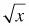2.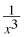3.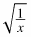4.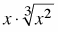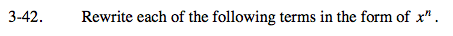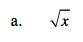The exponent, n, will be a fraction.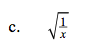Combine hints from part (a) and part (b).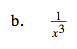The exponent, n, will be negative.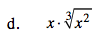$\text{Recall that }x=x^1=x^{\frac{3}{3}}$

$x\frac{5}{3}$